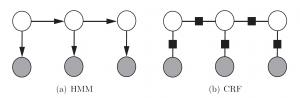0
0

浅谈中文分词

isnowfy 发表于 2014年04月18日 23:00 | Hits: 2478
Tag: algorithm | program | ir | math | nlpNLP（Natural language processing）自然语言处理一直都是比较热门的领域，现在不管是搜索，推荐神马的基本都需要和nlp打交道，而中文的nlp处理的第一步就是分词了，所以中文分词一直扮演者举足轻重的角色。当然了，分词的算法也是层出不穷，从最初的字典匹配到后来的统计模型，从HMM到CRF，分词精度都在不断提高，下面我就简单介绍下基本的分词算法。

统计模型

$$argmax\Pi_{i=1}^{m}{P(A_i)}$$

$$argmax\Pi{P(A_i|A_{i-1},A_{i-2}...,A_{i-n+1})}$$

隐马尔可夫模型HMM（Hidden Markov Model）

HMM隐藏马尔可夫链模型就是这样一个字标注的分词算法，假设原来的句子序列是$$a_1a_2a_3...a_n$$，那么HMM是要求这样的式子

$$argmax\Pi{P(c_i|c_{i-1})*P(a_i|c_i)}$$

最大熵模型ME（Maximum Entropy）

$$\overline{p}(f)=\Sigma{\overline{p}(a_i,c_i)*f(a_i,c_i)}=p(f)=\Sigma{p(c_i|a_i)*\overline{p}(a_i)*f(a_i,c_i)}$$

$$argmaxH(c_i|a_i)$$

H就是信息熵的函数，于是这样我们就求出了$$P(c_i|a_i)$$，就知道了每个字a的标注c了，最大熵模型的一个好处是我们可以引入各种各样的feature，而不仅仅是从字出现的频率去分词，比如我们可以加入domain knowledge，可以加入已知的字典信息等。

最大熵马尔可夫模型MEMM（Maximum-entropy Markov model）

$$argmax\Pi{P(c_i|c_{i-1},a_i)}$$

条件随机场CRF（Conditional Random Field）

MEMM的不足之处就是马尔可夫链的不足之处，马尔可夫链的假设是每个状态只与他前面的状态有关，这样的假设显然是有偏差的，所以就有了CRF模型，使得每个状态不止与他前面的状态有关，还与他后面的状态有关，从最开始的图片也能看出，HMM是基于贝叶斯网络的有向图，而CRF是无向图。

$$P(Y_v|Y_w,w \neq v)=P(Y_v,Y_w,w \sim v)$$where w~v means that w and v are neighbors in G.

$$P(y|x,\lambda)=\frac{1}{Z(x)}*exp(\Sigma{\lambda_j*F_j(y,x)})$$